Courses

# Quant Mock Test - 10

## 20 Questions MCQ Test Mock Test Series for CLAT 2020 | Quant Mock Test - 10

Description
This mock test of Quant Mock Test - 10 for CLAT helps you for every CLAT entrance exam. This contains 20 Multiple Choice Questions for CLAT Quant Mock Test - 10 (mcq) to study with solutions a complete question bank. The solved questions answers in this Quant Mock Test - 10 quiz give you a good mix of easy questions and tough questions. CLAT students definitely take this Quant Mock Test - 10 exercise for a better result in the exam. You can find other Quant Mock Test - 10 extra questions, long questions & short questions for CLAT on EduRev as well by searching above.
QUESTION: 1

### The area of a rectangle is equal to the area of a circle whose radius is 28 cm. If the ratio of the length to the breadth of the rectangle is 14 : 11, what is its perimeter?

Solution:

Area of circle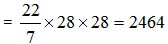Area of rectangle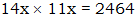x2 = 16
x = 4
Perimeter = (14+11) x 4 x 2
= 200.

QUESTION: 2

### Two dice are thrown together and the sum of the digits observed comes to 10. What is the probability of showing 4 on one of the dice?

Solution:

2 cases are there= (4, 6) (6, 4)
2/36 = 1/18

QUESTION: 3

### A man swims downstream from one point to another which is 6 km apart in 1.5 hours. It covers the same distance upstream in 2 hours. Find the speed of the man in still water.

Solution:

downstream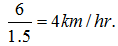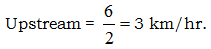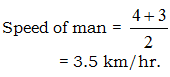QUESTION: 4

Find the missing number if the average of all the eight numbers is 714.

827   232   465   968   _____341   476   599

Solution:
QUESTION: 5

What will come in place of both question marks (?) in the following equation?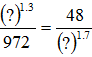Solution:

?3 = 48 x 972
?3 = 46656
? = 36

QUESTION: 6

The salary of a person is Rs 15,000. After 6 months it was increased by 25% and after another 3 months it was decreased by 15%. Find the salary of the person after 9 months.

Solution:

15000 x 1.25 x 0.85 = 15937.5

QUESTION: 7

In an election there were two candidates - Sushma and Sonia. The total number of votes in their constituency was 30,000, and 70% of the total votes were polled. If 40% of the votes were received by Sushma. Find number of votes received by Sonia?

Solution:

60% of 70% of 30,000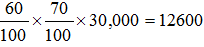QUESTION: 8

The ratio of two numbers is 3:7. If each number is increased by 10, the ratio becomes 7 : 13. Find the difference of the two numbers.

Solution: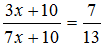39x + 130 = 49x + 70
60 = 10x
6 = x
42, 18
Diff = 24 Ans.

QUESTION: 9

The price of two bats and three balls is Rs 668 and the price of five bats and four balls is Rs 1544. What is the cost of one ball?

Solution:

[2 Bat + 3 Ball = 668] x 5
2[5 Bat + 4 Ball = 1544] x 2
10 Bat + 15 Ball = 3340
10 Bat + 8 Ball = 3088
7 Ball = 252
1 Ball = 36

QUESTION: 10

60 per cent of 2/7 of three-fourths of a number is 1836. What is the number?

Solution: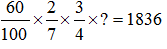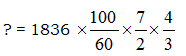= 14280.

QUESTION: 11

A TV when sold for Rs 7038 earned a profit of 15%. What was its cost price?

Solution:

C.P x 115% = 7038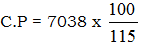= 6120

QUESTION: 12

An amount was distributed among A, B and C in the ratio of 3 : 5 : 7. If A received Rs 796 less than C, then what was the share of B?

Solution:

7x – 3x = 796
4x = 796
X = 199
5 x 199 = 995

QUESTION: 13

A can do a piece of work in 6 days, and B can do it in 18 days. How long will they take if both work together?

Solution: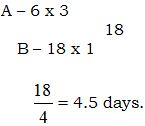QUESTION: 14

A boat covers a distance of 135 km downstream in 9 hours. To cover the same distance upstream, the boat takes 6 hours longer. What is the speed of the man in still water?

Solution: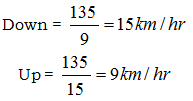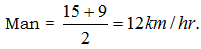QUESTION: 15

Directions: What should come in place of question mark (?) in the following questions?

Q.

40% of 60% of 3/5 of 2750 = ?

Solution: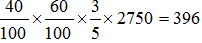QUESTION: 16

134.27 – 48.76 + 519.08 – 178.60 = ?

Solution:

425.99

QUESTION: 17

8.4 x 6.5 + 4.6 x 11.5 = ? ÷ 4

Solution: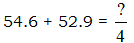430 = ?

QUESTION: 18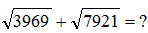Solution:

63 + 89
= 152

QUESTION: 19

42% of ? = 4116

Solution: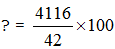= 9800

QUESTION: 20

If the students of a school are made to stand in a column of 64 each then 25 columns could be formed. If 40 students are made to stand in each column how many columns could be formed?

Solution:

64 x 25 = 40 x ?
? = 40.